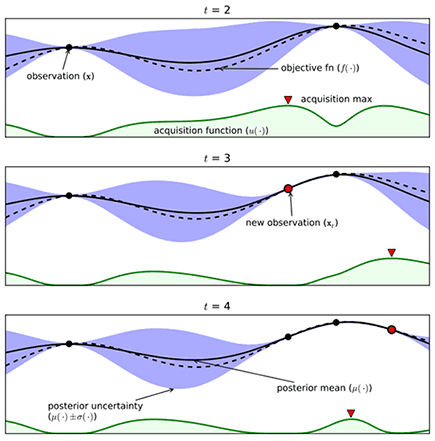This is part of our commercial offering.## V1Bayes

``polyaxon.polyflow.matrix.bayes.V1Bayes(kind='bayes', params=None, num_initial_runs=None, max_iterations=None, utility_function=None, metric=None, seed=None, concurrency=None, tuner=None, early_stopping=None)``

Bayesian optimization is an extremely powerful technique. The main idea behind it is to compute a posterior distribution over the objective function based on the data, and then select good points to try with respect to this distribution.

The way Polyaxon performs bayesian optimization is by measuring the expected increase in the maximum objective value seen over all experiments in the group, given the next point we pick.

• Args:
• kind: string, should be equal to `bayes`
• utility_function: UtilityFunctionConfig
• num_initial_runs: int
• max_iterations: int
• metric: V1OptimizationMetric
• params: List[Dict[str, params]]
• seed: int, optional
• concurrency: int, optional
• tuner: V1Tuner, optional
• early_stopping: List[EarlyStopping], optional

## YAML usage

``````matrix:
kind: bayes
utilityFunction:
numInitialRuns:
maxIterations:
metric:
params:
seed:
concurrency:
tuner:
earlyStopping:``````

## Python usage

``````from polyaxon import types
from polyaxon.polyflow import (
V1Bayes, V1HpLogSpace, V1HpChoice, V1FailureEarlyStopping, V1MetricEarlyStopping,
V1OptimizationMetric, V1Optimization, V1OptimizationResource, UtilityFunctionConfig
)
matrix = V1Bayes(
concurrency=20,
utility_function=UtilityFunctionConfig(...),
num_initial_runs=40,
max_iterations=20,
params={"param1": V1HpLogSpace(...), "param2": V1HpChoice(...), ... },
metric=V1OptimizationMetric(name="loss", optimization=V1Optimization.MINIMIZE),
early_stopping=[V1FailureEarlyStopping(...), V1MetricEarlyStopping(...)]
)``````

## Fields

### kind

The kind signals to the CLI, client, and other tools that this matrix is bayes.

If you are using the python client to create the mapping, this field is not required and is set by default.

``````matrix:
kind: bayes``````

### params

A dictionary of `key -> value generator` to generate the parameters.

To learn about all possible params generators.

The parameters generated will be validated against the component’s inputs/outputs definition to check that the values can be passed and have valid types.

``````matrix:
kind: bayes
params:
param1:
kind: ...
value: ...
param2:
kind: ...
value: ...``````

### utilityFunction

the utility function defines what acquisition function and bayesian process to use.

### Acquisition functions

A couple of acquisition functions can be used: `ucb`, `ei` or `poi`.

• `ucb`: Upper Confidence Bound,
• `ei`: Expected Improvement
• `poi`: Probability of Improvement

When using `ucb` as acquisition function, a tunable parameter `kappa` is also required, to balance exploitation against exploration, increasing kappa will make the optimized hyperparameters pursuing exploration.

When using `ei` or `poi` as acquisition function, a tunable parameter `eps` is also required, to balance exploitation against exploration, increasing epsilon will make the optimized hyperparameters more spread out across the whole range.

### Gaussian process

Polyaxon allows to tune the gaussian process.

• `kernel`: `matern` or `rbf`.
• `lengthScale`: float
• `nu`: float
• `numRestartsOptimizer`: int
``````matrix:
kind: bayes
utility_function:
acquisitionFunction: ucb
kappa: 1.2
gaussianProcess:
kernel: matern
lengthScale: 1.0
nu: 1.9
numRestartsOptimizer: 0``````

### numInitialRuns

the initial iteration of random experiments is required to create a seed of observations.

This initial random results are used by the algorithm to update the regression model for generating the next suggestions.

``````matrix:
kind: bayes
numInitialRuns: 40``````

### maxIterations

After creating the first set of random observations, the algorithm will use these results to update the regression model and suggest a new experiment to run.

Every time an experiment is done, the results are used as an observation and are appended to the historical values so that the algorithm can use all the observations again to suggest more experiments to run.

The algorithm will keep suggesting more experiments and adding their results as an observation, every time we make a new observation, i.e. an experiment finishes and reports the results to the platform, the results are appended to the historical values, and then used to make a better suggestion.

``````matrix:
kind: bayes
maxIterations: 15``````

This configuration will make 15 suggestions based on the historical values, every time an observation is made is appended to the historical values to make better subsequent suggestions.

### metric

The metric to optimize during the iterations, this is the metric that you want to maximize or minimize.

``````matrix:
kind: bayes
metric:
name: loss
optimization: minimize``````

### seed

Since this algorithm uses random generators, if you want to control the seed for the random generator, you can pass a seed.

``````matrix:
kind: bayes
seed: 523``````

### concurrency

An optional value to set the number of concurrent operations.

This value only makes sense if less or equal to the total number of possible runs.
``````matrix:
kind: bayes
concurrency: 20``````

### earlyStopping

A list of early stopping conditions to check for terminating all operations managed by the pipeline. If one of the early stopping conditions is met, a signal will be sent to terminate all running and pending operations.

``````matrix:
kind: bayes
earlyStopping: ...``````

For more details please check the early stopping section.

### tuner

The tuner reference (w/o component hub reference) to use. The component contains the logic for creating new suggestions based on bayesian optimization, users can override this section to provide a different tuner component.

``````matrix:
kind: bayes
tuner:
hubRef: 'acme/my-bo-tuner:version'``````

## Example

This is an example of using bayesian search for hyperparameter tuning:

``````matrix:
kind: bayes
concurrency: 5
maxIterations: 15
numInitialTrials: 30
metric:
name: loss
optimization: minimize
utilityFunction:
acquisitionFunction: ucb
kappa: 1.2
gaussianProcess:
kernel: matern
lengthScale: 1.0
nu: 1.9
numRestartsOptimizer: 0
params:
lr:
kind: uniform
value: [0, 0.9]
dropout:
kind: choice
value: [0.25, 0.3]
activation:
kind: pchoice
value: [[relu, 0.1], [sigmoid, 0.8]]
component:
inputs:
- name: batch_size
type: int
isOptional: true
value: 128
- name: lr
type: float
- name: dropout
type: float
container:
image: image:latest
command: [python3, train.py]
args: [
"--batch-size={{ batch_size }}",
"--lr={{ lr }}",
"--dropout={{ dropout }}",
"--activation={{ activation }}"``````